CiteScore 2.0
More on impact ›

# Frontiers in Applied Mathematicsand Statistics## ORIGINAL RESEARCH article

Front. Appl. Math. Stat., 11 February 2019 | https://doi.org/10.3389/fams.2019.00008

# Filtering Suppresses Amplitude Chimeras

• 1Chaos and Complex Systems Research Laboratory, Department of Physics, University of Burdwan, Burdwan, India
• 2Institut für Theoretische Physik, Technische Universität Berlin, Berlin, Germany

Amplitude chimera (AC) is an interesting chimera pattern that has been discovered recently and is distinct from other chimera patterns, like phase chimeras and amplitude mediated phase chimeras. Unlike other chimeras, in the AC pattern all the oscillators have the same phase velocity, however, the oscillators in the incoherent domain show periodic oscillations with randomly shifted origin. In this paper we investigate the effect of local filtering in the coupling path on the occurrence of AC patterns. Our study is motivated by the fact that in the practical coupling channels filtering effects come into play due to the presence of dispersion and dissipation. We show that a low-pass or all-pass filtering is actually detrimental to the occurrence of AC. We quantitatively establish that with decreasing cut-off frequency of the filter, an AC transforms into a synchronized pattern. We also show that the symmetry-breaking steady state, i.e., the oscillation death state can be revoked and rhythmogenesis can be induced by local filtering. Our study will shed light on the understanding of many biological systems where spontaneous symmetry-breaking and local filtering occur simultaneously.

## 1. Introduction

Networks of coupled identical oscillators show various cooperative behaviors. From the symmetry considerations they can be categorized into two broad types: (i) symmetric (or symmetry preserving) states, like synchronization, phase locking, and amplitude death (AD) state [1, 2], and (ii) symmetry-breaking states, such as oscillation death (OD)  and chimera states . Among all these cooperative behaviors, in the center of recent research is the chimera state [4, 5] discovered by Kuramoto and Battoghtokh in 2002. Chimera is a counterintuitive spatiotemporal pattern in which coherence and incoherence coexist in a network of identical oscillators [3, 6]. In the initial years studies on chimeras focused on exploring several aspects of chimera theoretically (see two recent reviews on chimeras in [3, 6] for a detailed discussion). Later on experimental observations of chimeras established their robustness in real systems. After the first experimental evidence of chimeras in optical systems  and chemical oscillators , they have been observed experimentally in several other systems also, e.g., in mechanical systems [9, 10], electronic [11, 12], optoelectronic delayed-feedback , electrochemical  oscillator systems and Boolean networks . Studies on chimeras are continuing to be a vibrant area of research owing to its connection to various natural phenomena and systems, including epileptic seizure , unihemispheric sleep [22, 23], ecological synchrony [24, 25], social systems , and quantum systems .

Although chimeras were discovered in phase oscillators, later on the notion was extended to the general class of oscillators having both phase as well as amplitude dynamics. Those oscillators may show amplitude mediated phase chimeras (AMC) , which is the coexistence of synchrony and asynchrony in both phase and amplitude: here in the incoherent (coherent) domain oscillators have disparate (same) phase velocities. Recently, a new type of chimera has been discovered by Zakharova et al.  called amplitude chimera (AC), in which all the oscillators of the network are correlated in phase, however, in the incoherent domain nodes have uncorrelated amplitude. The distinct signature of an AC state is that in its coherent domain nodes oscillate around the origin and have equal amplitude, however, nodes belonging to the incoherent domain show limit cycles of disparate amplitude and those limit cycles are shifted from the origin.

In contrast to other chimera patterns, AC has strong connections to another symmetry-breaking steady state, namely the oscillation death state (OD) [2, 3034]. The bridge between AC and OD is mediated by an interesting emergent spatial pattern called chimera death [29, 35], which carries the attributes of both AC and OD. Since AC is the coexistence of spatially homogeneous and inhomogeneous limit cycles, therefore, it is believed to have relevance in the underlying mechanism for cellular differentiation [36, 37] and ecological oscillations [24, 25, 38, 39] where coexistence of inhomogeneity and homogeneity appears naturally.

As amplitude chimeras are a recently discovered variant of chimera patterns, therefore, it is less explored: the effect of node dynamics and coupling on the occurrence of AC demands further investigations. Specifically, in realistic networks, where signals often suffer from time delay , noise, dispersion and dissipation , their effect on the AC pattern will be important to explore. Although, the effect of noise and time delay has recently been explored in detail in Loos et al.  and Gjurchinovski et al. , however, the effect of dispersion and dissipation on the AC state has not been studied yet. In the presence of dispersion, signals having different frequencies propagate with different velocities. Whereas, dissipation causes attenuation and signal loss. A channel having both dispersion and dissipation is said to behave like a low-pass filter. On the other hand, a channel having only dispersion is said to behave as an all-pass filter . Several physical and biological systems contain inherent local low-pass filters (LPFs): For example, the musculoskeletal system of human body acts as low-pass filter , the abdominal ganglion of crayfish contains local LPFs , LPF is one of the building blocks of phase-locked loops . On the other hand, in the case of electronic communications and neuronal systems the presence of local amplifiers or ion channels , respectively, compensate for the dissipation, however, in those systems signals still suffer dispersions making the coupling path to behave as an all-pass filter (APF). The effect of low-pass filtering was studied before in the context of synchronization [49, 50] and rhythmogenesis from an amplitude or oscillation death state  (by rhythmogenesis we mean the process by which the rhythmic behavior of individual nodes in a network of coupled oscillators is restored from the state of suppressed oscillations without changing the intrinsic parameters associated with the individual nodes); Banerjee et al.  reported a novel transition from homogeneous to inhomogeneous limit cycle as a consequence of low-pass or all-pass filtering. However, hitherto the effect of filtering on the chimera state in coupled oscillators has not been explored.

Motivated by the above discussion, in this paper we study the effect of local filtering on the occurrence of amplitude chimera (AC) in a network of nonlocally coupled Stuart-Landau oscillators. By local filtering we mean that the filtering effect is considered in the self-feedback path only. We consider local low-pass and all-pass filters in the network and for the first time we show that both types of filtering have a detrimental effect on the occurrence of amplitude chimeras: filtering always suppresses amplitude chimeras. With the variation of a filtering parameter (namely, the corner or cut-off frequency) we observe transitions from the oscillation death and amplitude chimera state to the globally synchronized state.

## 2. Without Filtering

We consider N = 200 Stuart-Landau oscillators interacting through nonlocal symmetry-breaking coupling (i.e., only through the x-variable). The mathematical model of the coupled system is given by,

with i = 1⋯200. The individual Stuart-Landau oscillators have unit amplitude and eigenfrequency ω. Here ε denotes the coupling strength and P is the coupling range of the nonlocal coupling.

To explore the dynamics of the coupled network we numerically solve Equation (1) using the fourth-order Runge-Kutta method (step size = 0.01). Throughout this paper we consider ω = 2 and use the following initial conditions : xi = 1 and yi = −1 for $1\le i\le \frac{N}{2}$ and xi = −1 and yi = 1 for $\frac{N}{2}.

Figure 1A shows the phase diagram in the P − ε space: we can see that the amplitude chimera (AC) state is interspersed in between the completely synchronized oscillation zone (Sync) and the oscillation death (OD) zone. This is in accordance with the results of Zakharova et al. , Schneider et al. , Zakharova et al. , and Tumash et al.  where this system was studied in detail. Figures 1B–D illustrate the spatiotemporal evolution of the synchronized state (ε = 5), AC pattern (ε = 20) and multicluster OD state (ε = 30) at P = 10. Figure 2 depicts the manifestation of AC and OD in the phase space for an exemplary coupling range P = 10. Figure 2 (Left panel) shows AC for ε = 20: here the small amplitude and shifted-origin limit cycles represent incoherent nodes and those having large amplitude oscillating around the origin represent the coherent nodes (for clarity only a few nodes from coherent and incoherent domains are shown). For higher coupling strengths a symmetry-breaking steady state (OD state) emerges, which is shown in Figure 2 (Right panel) for ε = 30. In the next section we will explore how filtering affects this dynamical landscape in parameter space.

FIGURE 1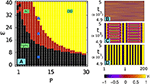Figure 1. Without filtering: (A) phase diagram in the P − ε space for N = 200 nonlocally coupled Stuart-Landau oscillators (ω = 2). Sync, synhronized state; AC, amplitude chimera; OD, oscillation death. (B–D) The spatiotemporal plots at P = 10 for three different coupling strengths ε: (B) synchronized state for ε = 5 [shown byin (A)], (C) AC for ε = 20 [shown byin (A)] and (D) multicluster OD for ε = 30 [shown inin (A)].

FIGURE 2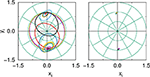Figure 2. Without filtering: Phase-space plot of a few nodes of the network from the coherent and incoherent domains (Left panel) AC (ε = 20,point in Figure 1A), and (Right panel) OD (ε = 30,point of Figure 1A). Other parameter values are P = 10, ω = 2, N = 200.

## 3. Effect of Low-Pass Filtering

### 3.1. Mathematical Model

We consider N = 200 Stuart-Landau oscillators interacting through nonlocal symmetry-breaking coupling as in Equation (1), but here we consider local low-pass filter in the coupling path. The mathematical model of the coupled system is given by,

Equation ((2c)) is the mathematical equation of a low-pass filter whose input is xi and output is zi. This zi is fed to the coupling part of Equation (2a). Here α represents the corner or cut-off frequency of the LPF: the lower is the value of α, the higher is the effect of filtering. For larger α, filtering effects become lesser: if we put α → ∞ in Equation (2a), it simply gives zi = xi, i.e., no filtering effect is present and Equation (2) reduces to the original Equation (1). Since in the literature of filters we are conversant with the frequency domain representation, therefore, at first it is difficult to realize the role of α in Equation (2c). However, a close inspection reveals that α controls both phase and amplitude of the output signal zi by the following way: the phase shift between input and output is given by ${\varphi }_{i}=arctan\left(\omega {\alpha }^{-1}\right)$, the ratio of output and input (called gain of the filter) is $G=\frac{1}{\sqrt{1+{\omega }^{2}{\alpha }^{-2}}}$ (see  for details). Another equivalent form is the representation of Equation (2c) as a distributed delayed coupling term in Equation (2a) with an exponential delay kernel exp(−ατ) [55, 56].

### 3.2. Results

We investigate the effect of local low-pass filtering on the occurrence of amplitude chimera. Since α is the only control parameter, we will explore the effect of α on the dynamics of the network. We keep all the parameters and initial conditions the same as in the unfiltered case; the initial conditions for the filter variable zi are chosen the same as those of xi for the unfiltered case.

Figures 3A,B demonstrate the phase diagram of the network in the P − ε space for three different (decreasing) values of α. It can be observed from Figure 3 that the smaller the value of α is, the more the network dynamics deviates from the original scenario shown in Figure 1A. It is apparent from Figure 3 that with decreasing α (i.e., increasing filtering effect) the synchronized portion dominates and therefore suppresses the AC and OD regions: a lower α shifts the AC and OD zone to a higher P region and also quenches the area of the AC and OD zone. Eventually, below a critical value of α (say αc) the AC and OD state disappear and only the synchronized state prevails in the whole P − ε space. This suppression of the AC and OD zone is shown in Figure 3C for α = 10.

FIGURE 3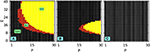Figure 3. With local low-pass filtering: Phase diagram in the P vs ε space (N = 200, ω = 2) for (A) α = 50 and (B) α = 20. (C) shows complete suppression of AC for α = 10.

The scenario can be understood more clearly in the ε − α space for a fixed P. Figure 4A shows this for P = 10: we can observe that for comparatively high values of α the dynamics of the system remains unchanged. However, as the value of α is decreased the system goes to a synchronized state irrespectively of ε. It also shows that there exists a critical value αc of α, below which the synchronized state is the only possible state. Figures 4B–D illustrate how decreasing α leads to the transition from OD to synchrony via AC (ε = 25 and P = 10): for α = 45 the network shows a multi-clustered OD state (Figure 4B), and the AC state is shown for α = 35 (Figure 4C), and finally global synchrony (a coherent traveling wave or a splay state) appears for further lowering of α (Figure 4D for α = 25). It is noteworthy that in a range of lower ε, no OD state occurs and in this zone a decreasing α leads to a direct transition from AC to synchrony.

FIGURE 4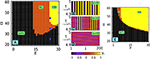Figure 4. With local low-pass filtering: (A) phase diagram in the ε − α space for P = 10 (ω = 2). Three points at three α values at a particular ε = 25 are marked by(α = 45),(α = 35) and(α = 25). (B–D) spatiotemporal plots corresponding to those three points (decreasing α): (B) multicluster OD, (C) AC, (D) synchronized state (coherent traveling wave or splay state). (E) phase diagram in the P − α space for ε = 35.

Figure 4E shows the scenario in the P − α space (ε = 35): here also we can see that at lower α values the completely synchronized state emerges out of either AC or OD. From Figures 4A,E we see that OD state is predominant for higher coupling strength (ε) and near-global coupling range (i.e., PN/2): it is interesting to note that a suitably chosen filtering parameter α can suppress the steady state and therefore results in rhythmogenesis in the network. In Zou et al.  and Banerjee et al.  filtering-induced rhythmogenesis in coupled oscillators was reported, however, in contrast to Zou et al.  and Banerjee et al.  here we show the existence of a broad parameter zone where OD does not transform into oscillation (SYNC) directly, but another symmetry-breaking emergent state, i.e., an amplitude chimera, mediates the transition. Therefore, filtering plays an important role in networks of physical, biological, and physiological systems where the occurrence of oscillation suppression often leads to a fatal system degradation and an irrecoverable malfunctioning . A similar enhancement of the stability domain of the synchronized solutions for small α was found for distributed delayed coupling with an exponential kernel .

In the above results we use suitable measures, such as the measure of spatial correlation (g0) and the center of mass (ycmi) to ensure the occurrence of the synchronized state and AC state and also to distinguish them (distinction of the OD state is relatively simple as we have to check whether a steady state is reached or not). According to Kemeth et al. , the measure of spatial correlation is defined in terms of the normalized probability density function g as

Here $\stackrel{^}{L}{\psi }_{i}\left(t\right)$ represents the local curvature at each node i at time t given by

where $\stackrel{^}{L}$ is the discrete Laplacian operator on each snapshot {ψi}. In our present case the state variable ψi(t) = yi (one can use xi as well). In Equation (3) we consider a threshold value δth = 0.01Lmax, where Lmax is the maximum curvature in the network . The measure of spatial correlation g0(t) = 1 for a fully synchronized network and g0(t) = 0 for a completely unsynchronized network. Therefore, 0 < g0(t) < 1 represents partial synchronization ensuring the occurrence of chimera state. Although g0(t) can ensure the occurrence of a chimera state, it cannot distinguish between phase and amplitude chimeras. To ensure that AC indeed emerges in the network, we compute the center of mass of each oscillator defined by 

where yi represents the state of the i-th oscillator and T is a sufficiently large time. The quantity ycmi gives a measure of the shift of a limit cycle from the origin. Therefore, it can distinguish the homogeneous limit cycles from inhomogeneous ones.

Figures 5A,C, respectively, show g0(t) and ycmi of each oscillator corresponding to the synchronized state of Figure 4D (α = 25): we observe that all the oscillators in the network have g0(t) = 1 and ycmi = 0 indicating that the whole network is synchronized. On the other hand, Figures 5B,D, respectively, show g0(t) and ycmi corresponding to the AC state of Figure 4C (α = 35): we can see that 0 < g0(t) < 1 indicating the occurrence of chimeras and at the same time ycmi in the incoherent region exhibits a random sequence of shifts to positive and negative values, however, in the coherent region ycmi = 0 indicating that the resulting chimera is indeed an AC pattern.

FIGURE 5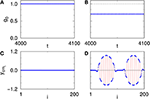Figure 5. With local low-pass filtering: (A,B) The time evolution of g0 corresponding to the synchronized (A) and AC (B) state as marked in Figure 4A by () (α = 25) and () (α = 35), respectively. (C,D) The corresponding center of mass (ycmi) for the above two points, showing synchronized (C) and AC (D) states, respectively. Other parameters are P = 10, ε = 25 and ω = 2.

According to Tumash et al. , a strong measure that distinguishes an AC state from the synchronized state is the Floquet exponent. We study the stability of the periodic solution of nonlocally coupled Stuart-Landau oscillators given by (2) using Floquet theory . We rewrite (2) as

with x(t) ∈ Rn and also consider that a periodic solution ψ(t) = ψ(t + T) exists. In our case, we have three equations, therefore, n = 3N. The linearized equation is written as,

where J(ψ(t)) is the Jacobian matrix evaluated at ψ(t) and has the following solution:

Here δx(0) is the initial condition. The fundamental matrix M(t) obeys the equation,

where M(0) = 1, and M(t + T) = M(t)M(T). M(T) is the monodromy matrix whose eigenvalues are called Floquet multipliers (μk). Each Floquet multiplier can be expressed as μk = exp((Λk + k)T), where (Λk + k) is the Floquet exponent. The stability of the periodic orbit can be analyzed by determining the sign of the real part of these exponents. When the real parts of all the Floquet exponents are less than zero (i.e., Λk < 0) except the Goldstone mode (which is equal to zero) then the periodic solution is stable indicating a synchronized solution . But according to Tumash et al.  when at least one or two of them are greater than zero (Λk > 0), then the solution becomes unstable indicating a saddle cycle in phase space which corresponds to an AC state. In our computation we average the exponents over 200T (where T = π). Figure 6 shows the zone in black where all the exponents are negative (except the Goldstone mode), which indicates the synchronized state; Again, at every point in the orange region, a few Λks have small (< 0.5) positive values, which means that the system is in the AC state. Note the agreement between Figure 4A and Figure 6, which confirms that a transition from AC to synchrony indeed occurs with decreasing α.

FIGURE 6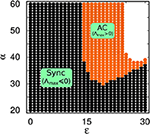Figure 6. With local low-pass filtering: Phase diagram of the periodic solutions (Sync and AC) in ε − α space based on the Floquet exponent. For the synchronized region (black), at each point, the largest real part of the Floquet exponents (Λmax) is negative (for the Goldstone mode it is approximately equal to zero). For the AC region (orange) at each point it is greater than zero (i.e., Λmax > 0). Other parameters are P = 10, ω = 2, N = 200.

## 4. Effect of All-Pass Filtering

Next we consider the effect of all-pass filtering (APF) in the network of Stuart-Landau oscillators described in Equation (1). The mathematical model of the coupled system is given by

Equations (10c, 10d) jointly represent the differential algebraic equation of an all-pass filter, whose input is xi and output is Ui . In this case α has the same meaning as in Equation (2c), but the effect of α is different on Ui: Here α does not affect the amplitude of Ui, it only affects the phase part by introducing a phase shift between the input and output signals, given by θ = 2arctan(ωα−1). Note that for the same α the phase shift introduced by a LPF (i.e., ϕ) is half of that of an APF (i.e., θ).

In Figure 7 the effect of an all-pass filter is shown in the P − ε space for two α values: Figure 7A is for α = 50 and Figure 7B is for α = 20. Figures 7C,D show the spatiotemporal representation of AC (for α = 50) and synchronized state (coherent traveling wave for α = 20), respectively: it shows that α acts as an efficient control parameter for the suppression of AC. Here it is evident that local all-pass filtering can also suppress AC (and OD) and gives rise to the synchronized state. Comparing Figures 7A,B of the low-pass filtering case with Figures 7A,B, respectively, it is interesting to note that for the same α an APF is more effective than a LPF as far as the suppression of amplitude chimeras (and OD) is concerned: see for example at α = 20, low-pass filtering only quenches the AC and OD zone in P − ε space (Figure 3B), however, all-pass filtering completely suppresses the AC and OD zone (Figure 7B). We ensure that αc, the critical value below which AC and OD are completely suppressed, is much higher for an APF compared to that of a LPF (not shown here): therefore even a relatively weak all-pass local filtering is equivalent to a stronger local low-pass filtering, as far as suppressing AC and OD is concerned. This is the consequence of the fact that at a particular value of α, the phase shift introduced by an APF is twice of that of a LPF . Therefore, all the results suggest that α which is the control parameter of local filters, also controls the dynamics of the whole network.

FIGURE 7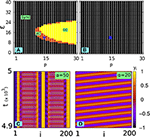Figure 7. With all-pass filtering: Phase diagram in the P − ε space for (A) α = 50 and (B) α = 20. (C) spatiotemporal plot of AC corresponding to α = 50 (shown byin A). (D) spatiotemporal plot of the synchronized state (coherent traveling wave) corresponding to α = 20 (shown byin B). In both (C,D) ε = 12, P = 15, ω = 2.

## 5. Conclusion

In this paper, we have revealed that the presence of local filtering (either low-pass or all-pass) suppresses the amplitude chimera state and therefore gives rise to global synchrony (coherent traveling waves). Further, it has been shown that local filtering causes rhythmogenesis by suppressing the steady state behavior (i.e., OD state), which has immense importance in many biological and engineering systems [58, 61]. Collectively, our study has a broad significance: it establishes that local filtering is detrimental for the symmetry-breaking states (AC and OD) and favors restoration of the symmetry in the network.

Our study reveals that the cut-off frequency α of the local filter acts as an efficient control parameter of the network that can be tuned to achieve a desired symmetry-breaking state or synchronized state without changing coupling strength or range. Several control methods to stabilize phase or amplitude mediated phase chimeras have recently been proposed . In Gjurchinovski et al.  it has been shown that a constant time delay in the coupling path can stabilize amplitude chimeras. In contrast, here we established that the local filtering has a destabilizing effect on the occurrence of amplitude chimeras. In the case of rhythmogenesis, the value of α that suppresses the steady state depends upon the system and coupling parameters in a nontrivial manner (see  for two mean-field coupled oscillators). It has been observed that if one wants to ensure rhythmogenesis (irrespectively of other parameters) the typical value of α is of the order of the intrinsic frequency of an individual oscillator (here ω). However, depending upon system and coupling parameters, α (filtering) need not be so small (strong): rhythmogenesis appears much before that, i.e., for α > ω.

From the perspective of dynamical systems the role of α can be understood in the following way: α actually controls the dissipative property of the whole network by controlling the dissipation and dispersion in the coupling path; a smaller α imposes a larger filtering effect and therefore smaller dissipation, which favors synchrony and rhythmogenesis. In this context we observe that filtering does not affect the pattern of phase chimera appreciably. This may be due to the fact that additional phase shift and/or attenuation caused by filtering has lesser effect on the mean frequency than on the amplitude dynamics (note that in the phase chimera the mean frequency is the determining factor that distinguishes the coherent and incoherent domains, whereas in the amplitude chimera, the amplitude of the nodes matters).

In this paper we have considered a network of Stuart-Landau oscillators. However, we verified that the filtering affects the amplitude chimera in a similar way in other systems also, for example, in a network of Rayleigh oscillators  (results not shown). Since the Stuart-Landau oscillator is a generic model for systems near a Hopf bifurcation and since filtering naturally arises in many biological and physical systems, we believe that our results can also be extended to those systems.

## Author Contributions

TB, ES, and AZ formulated the problem. BB and TB carried out the analysis. BB performed the computations. All authors discussed the results and contributed to writing the manuscript, read and approved the final manuscript.

## Conflict of Interest Statement

The authors declare that the research was conducted in the absence of any commercial or financial relationships that could be construed as a potential conflict of interest.

## Acknowledgments

BB acknowledges University of Burdwan for providing financial support through the state funded research fellowship. AZ and ES acknowledge the financial support by DFG in the framework of SFB 910.

## References

1. Pikovsky AS, Rosenblum MG, Kurths J. Synchronization. A Universal Concept In Nonlinear Science. Cambridge: Cambridge University Press (2001).

2. Koseska A, Volkov E, Kurths J. Oscillation quenching mechanisms: Amplitude vs oscillation death. Physics Reports (2013) 531:173. doi: 10.1016/j.physrep.2013.06.001

3. Schöll E. Synchronization patterns and chimera states in complex networks: Interplay of topology and dynamics. Eur Phys J Special Topics (2016) 225: 891. doi: 10.1140/epjst/e2016-02646-3

4. Kuramoto Y, Battogtokh D. Coexistence of coherence and incoherence in nonlocally coupled phase oscillators. Nonlin Phen Complex Sys. (2002) 5:380.

5. Abrams DM, Strogatz SH. Chimera states for coupled oscillators. Phys. Rev. Lett. 93 (2004):174102. doi: 10.1103/PhysRevLett.93.174102

6. Panaggio MJ, Abrams DM. Chimera states: Coexistence of coherence and incoherence in networks of coupled oscillators. Nonlinearity (2015) 28:R67–87. doi: 10.1088/0951-7715/28/3/R67

7. Hagerstrom AM, Murphy TE, Roy R, Hövel P, Omelchenko I, Schöll E. Experimental observation of chimeras in coupled-map lattices. Nat Phys. (2012) 8:658–61. doi: 10.1038/nphys2372

8. Tinsley MR, Nkomo S, Showalter K. Chimera and phase-cluster states in populations of coupled chemical oscillators. Nat Phys. (2012) 8:662–65. doi: 10.1038/nphys2371

9. Martens EA, Thutupalli S, Fourriere A, Hallatschek O. Chimera states in mechanical oscillator networks. Proc Nat Acad Sci USA. (2013) 110:10563–7. doi: 10.1073/pnas.1302880110

10. Kapitaniak T, P Kuzma JW, Czolczynski K, Maistrenko Y. Imperfect chimera states for coupled pendula. Sci Rep. (2014) 4:6379. doi: 10.1038/srep06379

11. Larger L, Penkovsky B, Maistrenko Y. Virtual chimera states for delayed-feedback systems. Phys Rev Lett. (2013) 111:054103. doi: 10.1103/PhysRevLett.111.054103

12. Gambuzza LV, Buscarino A, Chessari S, Fortuna L, Meucci R, Frasca M. Experimental investigation of chimera states with quiescent and synchronous domains in coupled electronic oscillators. Phys Rev E (2014) 90:032905. doi: 10.1103/PhysRevE.90.032905

13. Larger L, Penkovsky B, Maistrenko Y. Laser chimeras as a paradigm for multistable patterns in complex systems. Nat Commun. (2015) 6:7752. doi: 10.1038/ncomms8752

14. Hart JD, Bansal K, Murphy TE, Roy R. Experimental observation of chimeras in a minimal globally coupled network. Chaos (2016) 26:094801. doi: 10.1063/1.4953662

15. Hart J, Larger L, Murphy T, Roy R. Delayed dynamical systems: networks, chimeras and reservoir computing. arXiv 1808.04596 (2018).

16. Brunner D, Penkovsky B, Levchenko R, Schöll E, Larger L, Maistrenko Y. Two-dimensional spatiotemporal complexity in dual-delayed nonlinear feedback systems: chimeras and dissipative solitons. Chaos (2018) 28:103106. doi: 10.1063/1.5043391

17. Wickramasinghe M, Kiss IZ. Spatially organized dynamical states in chemical oscillator networks: synchronization, dynamical differentiation, and chimera patterns. PLoS ONE (2013) 8:e80586. doi: 10.1371/journal.pone.0080586

18. Wickramasinghe M, Kiss IZ. Spatially organized partial synchronization through the chimera mechanism in a network of electrochemical reactions. Phys Chem Chem Phys. (2014) 16:18360. doi: 10.1039/C4CP02249A

19. Schmidt L, Schönleber K, Krischer K, Garcia-Morales V. Coexistence of synchrony and incoherence in oscillatory media under nonlinear global coupling. Chaos (2014) 24:013102. doi: 10.1063/1.4858996

20. Rosin DP, Rontani D, Haynes ND, Schöll E, Gauthier DJ. Transient scaling and resurgence of chimera states in networks of boolean phase oscillators. Phys Rev E (2014) 90:030902(R). doi: 10.1103/PhysRevE.90.030902

21. Rothkegel A, Lehnertz K. Irregular macroscopic dynamics due to chimera states in small-world networks of pulse-coupled oscillators. New J Phys. (2014) 16:055006. doi: 10.1088/1367-2630/16/5/055006

22. Rattenborg NC, Amlaner CJ, Lima SL. Behavioral, neurophysiological and evolutionary perspectives on unihemispheric sleep. Neurosci Biobehav Rev. (2000) 24:817. doi: 10.1016/S0149-7634(00)00039-7

23. Rattenborg NC, Voirin B, Cruz SM, Tisdale R, Dell'Omo G, Lipp HP, et al. Evidence that birds sleep in mid-flight. Nat Commun. (2016) 7:12468. doi: 10.1038/ncomms12468

24. Banerjee T, Dutta PS, Zakharova A, Schöll E. Chimera patterns induced by distance-dependent power-law coupling in ecological networks. Phys Rev E (2016) 94:032206. doi: 10.1103/PhysRevE.94.032206

25. Dutta PS, Banerjee T. Spatial coexistence of synchronized oscillation and death: a chimeralike state. Phys Rev E (2015) 92:042919. doi: 10.1103/PhysRevE.92.042919

26. Gonzalez-Avella JC, Cosenza MG, Miguel MS. Localized coherence in two interacting populations of social agents. Physica A (2014) 399:24. doi: 10.1016/j.physa.2013.12.035

27. Bastidas VM, Omelchenko I, Zakharova A, Schöll E, Brandes T. Quantum signatures of chimera states. Phys Rev E (2015) 92:062924. doi: 10.1103/PhysRevE.92.062924

28. Sethia GC, Sen A, Johnston GL. Amplitude-mediated chimera states. Phys Rev E (2013) 88:042917. doi: 10.1103/PhysRevE.88.042917

29. Zakharova A, Kapeller M, Schöll E. Chimera death: symmetry breaking in dynamical networks. Phys Rev Lett. (2014) 112:154101. doi: 10.1103/PhysRevLett.112.154101

30. Zakharova A, Schneider I, Kyrychko YN, Blyuss KB, Koseska A, Fiedler B, et al. Time delay control of symmetry-breaking primary and secondary oscillation death. Europhys Lett. (2013) 104:50004. doi: 10.1209/0295-5075/104/50004

31. Banerjee T, Ghosh D. Transition from amplitude to oscillation death under mean-field diffusive coupling. Phys Rev E (2014) 89:052912. doi: 10.1103/PhysRevE.89.052912

32. Banerjee T, Ghosh D. Experimental observation of a transition from amplitude to oscillation death in coupled oscillators. Phys Rev E (2014) 89:062902. doi: 10.1103/PhysRevE.89.062902

33. Ghosh D, Banerjee T. Transitions among the diverse oscillation quenching states induced by the interplay of direct and indirect coupling. Phys Rev E (2014) 90:062908. doi: 10.1103/PhysRevE.90.062908

34. Ghosh D, Banerjee T, Kurths J. Mixed-mode oscillation suppression states in coupled oscillators. Phys Rev E (2015) 92:052913. doi: 10.1103/PhysRevE.92.052913

35. Banerjee T. Mean-field diffusion induced chimera death state. EPL (2015) 110:60003. doi: 10.1209/0295-5075/110/60003

36. Koseska A, Ullner E, Volkov E, Kurths J, Garcá-Ojalvo J. Cooperative differentiation through clustering in multicellular populations. J Theoret Biol. (2010) 263:189–202. doi: 10.1016/j.jtbi.2009.11.007

37. Chickarmane V, Troein C, Nuber UA, Sauro HM, Peterson C. Transcriptional dynamics of the embryonic stem cell switch. PLoS Comp Biol. (2006) 2:e123. doi: 10.1371/journal.pcbi.0020123

38. Banerjee T, Dutta PS, Gupta A. Mean-field dispersion-induced spatial synchrony, oscillation and amplitude death, and temporal stability in an ecological model. Phys Rev E (2015) 91:052919. doi: 10.1103/PhysRevE.91.052919

39. Arumugam R, Dutta P, Banerjee T. Environmental coupling in ecosystems: from oscillation quenching to rhythmogenesis. Phys Rev E (2016) 94:022206. doi: 10.1103/PhysRevE.94.022206

40. Biswas D, Banerjee T. Time-Delayed Chaotic Dynamical Systems. Springer International Publishing (2018).

41. Zou W, Zhan M, Kurths J. Revoking amplitude and oscillation deaths by low-pass filter in coupled oscillators. Phys Rev E (2017) 95:062206. doi: 10.1103/PhysRevE.95.062206

42. Loos SAM, Claussen JC, Schöll E, Zakharova A. Chimera patterns under the impact of noise. Phys Rev E (2016) 93:012209. doi: 10.1103/PhysRevE.93.012209

43. Gjurchinovski A, Schöll E, Zakharova A. Control of amplitude chimeras by time delay in dynamical networks. Phys Rev E (2017) 95:042218. doi: 10.1103/PhysRevE.95.042218

44. Sedra AS, Smith KC. Microelectronic Circuits. Oxford: Oxford University Press. (2003).

45. Nakashima AM, Borland MJ, Abel SM. Measurement of noise and vibration in canadian forces armoured vehicles. Ind Health (2007) 45:318. doi: 10.2486/indhealth.45.318

46. Stark L. Neurological Control Systems: Studies in Bioengineering. New York, NY: Plenum Press (1968).

47. Banerjee T, Paul B, Sarkar BC. Spatiotemporal dynamics of a digital phase-locked loop based coupled map lattice system. Chaos (2014) 24:013116. doi: 10.1063/1.4863859

48. Izhikevich E. Dynamical Systems in Neuroscience: The Geometry of Excitability and Bursting. Cambridge, MA: The MIT press (2007).

49. Kim MY, Sramek C, Uchida A, Roy R. Synchronization of unidirectionally coupled mackey-glass analog circuits with frequency bandwidth limitations. Phys Rev E (2006) 74:016211. doi: 10.1103/PhysRevE.74.016211

50. Soriano MC, Ruiz-Oliveras F, Colet P, Mirasso CR. Synchronization properties of coupled semiconductor lasers subject to filtered optical feedback. Phys Rev E (2008) 78:046218. doi: 10.1103/PhysRevE.78.046218

51. Banerjee T, Biswas D, Ghosh D, Bandyopadhyay B, Kurths J. Transition from homogeneous to inhomogeneous limit cycles: effect of local filtering in coupled oscillators. Phys Rev E (2018) 97:042218. doi: 10.1103/PhysRevE.97.042218

52. Schneider I, Kapeller M, Loos S, Zakharova A, Fiedler B, Schöll E. Stable and transient multi-cluster oscillation death in nonlocally coupled networks. Phys Rev E (2015) 92:052915. doi: 10.1103/PhysRevE.92.052915

53. Zakharova A, Kapeller M, Schöll E. Amplitude chimeras and chimera death in dynamical networks. J Phys Conf Ser. (2016) 727:012018. doi: 10.1088/1742-6596/727/1/012018

54. Tumash L, Zakharova A, Lehnert J, Just W, Schöll E. Stability of amplitude chimeras in oscillator networks. EPL (2017) 117:20001. doi: 10.1209/0295-5075/117/20001

55. Kyrychko YN, Blyuss KB and Schöll E. Amplitude death in systems of coupled oscillators with distributed-delay coupling. Eur Phys J B (2011) 84:307. doi: 10.1140/epjb/e2011-20677-8

56. Kyrychko YN, Blyuss KB, Schöll E. Synchronization of networks of oscillators with distributed-delay coupling. Chaos (2014) 24:043117. doi: 10.1063/1.4898771

57. Zou W, Senthilkumar DV, Zhan M, Kurths J. Reviving oscillations in coupled nonlinear oscillators. Phys Rev Lett. (2013) 111:014101. doi: 10.1103/PhysRevLett.111.014101

58. Zou W, Senthilkumar DV, Nagao R, Kiss IZ, Tang Y, Koseska A, et al. Restoration of rhythmicity in diffusively coupled dynamical networks. Nat Commun. (2015) 6:7709. doi: 10.1038/ncomms8709

59. Ghosh D, Banerjee T, Kurths J. Revival of oscillation from mean-field-induced death: theory and experiment. Phys Rev E (2015) 92:052908. doi: 10.1103/PhysRevE.92.052908

60. Kemeth FP, Haugland SW, Schmidt L, Kevrekidis IG, Krischer K. A classification scheme for chimera states. Chaos (2016) 26:094815. doi: 10.1063/1.4959804

61. Motter AE, Myers SA, Anghel M, Nishikawa T. Spontaneous synchrony in power-grid networks. Nat Phys. (2013) 9:191–7. doi: 10.1038/nphys2535

62. Sieber J, Omel'chenko O, Wolfrum M. Controlling unstable chaos: stabilizing chimera states by feedback. Phys Rev Lett. (2014) 112:054102. doi: 10.1103/PhysRevLett.112.054102

63. Bick C, Martens EA. Controlling chimeras. New J Phys. (2015) 17:033030. doi: 10.1088/1367-2630/17/3/033030

64. Omelchenko I, Omel'chenko OE, Zakharova A, Wolfrum M, Schöll E. Tweezers for chimeras in small networks. Phys Rev Lett. (2016) 116:114101. doi: 10.1103/PhysRevLett.116.114101

65. Banerjee T, Biswas D, Ghosh D, Schöll E, Zakharova A. Networks of coupled oscillators: from phase to amplitude chimeras. Chaos (2018) 28:113124. doi: 10.1063/1.5054181

Keywords: chimera, amplitude chimera, oscillation death, filtering, control, rhythmogenesis, all-pass filter

Citation: Banerjee T, Bandyopadhyay B, Zakharova A and Schöll E (2019) Filtering Suppresses Amplitude Chimeras. Front. Appl. Math. Stat. 5:8. doi: 10.3389/fams.2019.00008

Received: 30 November 2018; Accepted: 24 January 2019;
Published: 11 February 2019.

Edited by:

Ulrich Parlitz, Max-Planck-Institute for Dynamics and Self-Organisation, Max Planck Society (MPG), Germany

Reviewed by:

Isao T. Tokuda, Ritsumeikan University, Japan
Anastasiia Panchuk, Institute of Mathematics (NAN Ukraine), Ukraine

Copyright © 2019 Banerjee, Bandyopadhyay, Zakharova and Schöll. This is an open-access article distributed under the terms of the Creative Commons Attribution License (CC BY). The use, distribution or reproduction in other forums is permitted, provided the original author(s) and the copyright owner(s) are credited and that the original publication in this journal is cited, in accordance with accepted academic practice. No use, distribution or reproduction is permitted which does not comply with these terms.

*Correspondence: Tanmoy Banerjee, tbanerjee@phys.buruniv.ac.in
Eckehard Schöll, schoell@physik.tu-berlin.de